# How to Calculate and Solve for Thickness of Solidifying Metals | Solidification of Metals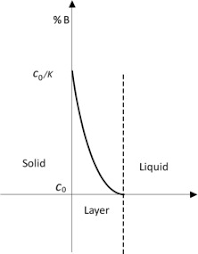The image above represents thickness of solidifying metals.

To compute for thickness of solidifying metals, six essential parameters are needed and these parameters are Melting Temperature of Metal (Tm), Initial Mould Temperature (To), Heat Diffusivity (α), Time (t), Density (ρ’) and Latent Heat of Fusion (Hf).

The formula for calculating thickness of solidifying metals:

M = 2(Tm – To)√(α)√(t) / √(π)ρ’Hf

Where:

M = Thickness of Solidifying Metal
Tm = Melting Temperature of Metal
To = Initial Mould Temperature
α = Heat Diffusivity
t = Time
ρ = Density
Hf = Latent Heat of Fusion

Let’s solve an example;
Find the thickness of solidifying metal when the melting temperature of metal is 4, the initial mould temperature is 8, the heat diffusivity is 2, the time is 6, the density is 3 and the latent heat of fusion is 7.

This implies that;

Tm = Melting Temperature of Metal = 4
To = Initial Mould Temperature = 8
α = Heat Diffusivity = 2
t = Time = 6
ρ = Density = 3
Hf = Latent Heat of Fusion = 7

M = 2(Tm – To)√(α)√(t) / √(π)ρ’Hf
M = 2(4 – 8)√(2)√(6) / √(π)(3)(7)
M = 2(-4)(1.414)(2.449) / (1.772)(3)(7)
M = -27.712 / 37.22
M = -0.744

Therefore, the thickness of solidifying metal is -0.744 m.

Nickzom Calculator – The Calculator Encyclopedia is capable of calculating the thickness of solidifying metals.

To get the answer and workings of the thickness of solidifying metals using the Nickzom Calculator – The Calculator Encyclopedia. First, you need to obtain the app.

You can get this app via any of these means:

To get access to the professional version via web, you need to register and subscribe for NGN 2,000 per annum to have utter access to all functionalities.
You can also try the demo version via https://www.nickzom.org/calculator

Apple (Paid) – https://itunes.apple.com/us/app/nickzom-calculator/id1331162702?mt=8
Once, you have obtained the calculator encyclopedia app, proceed to the Calculator Map, then click on Materials and Metallurgical under Engineering.Now, Click on Solidification of Metal under Materials and Metallurgical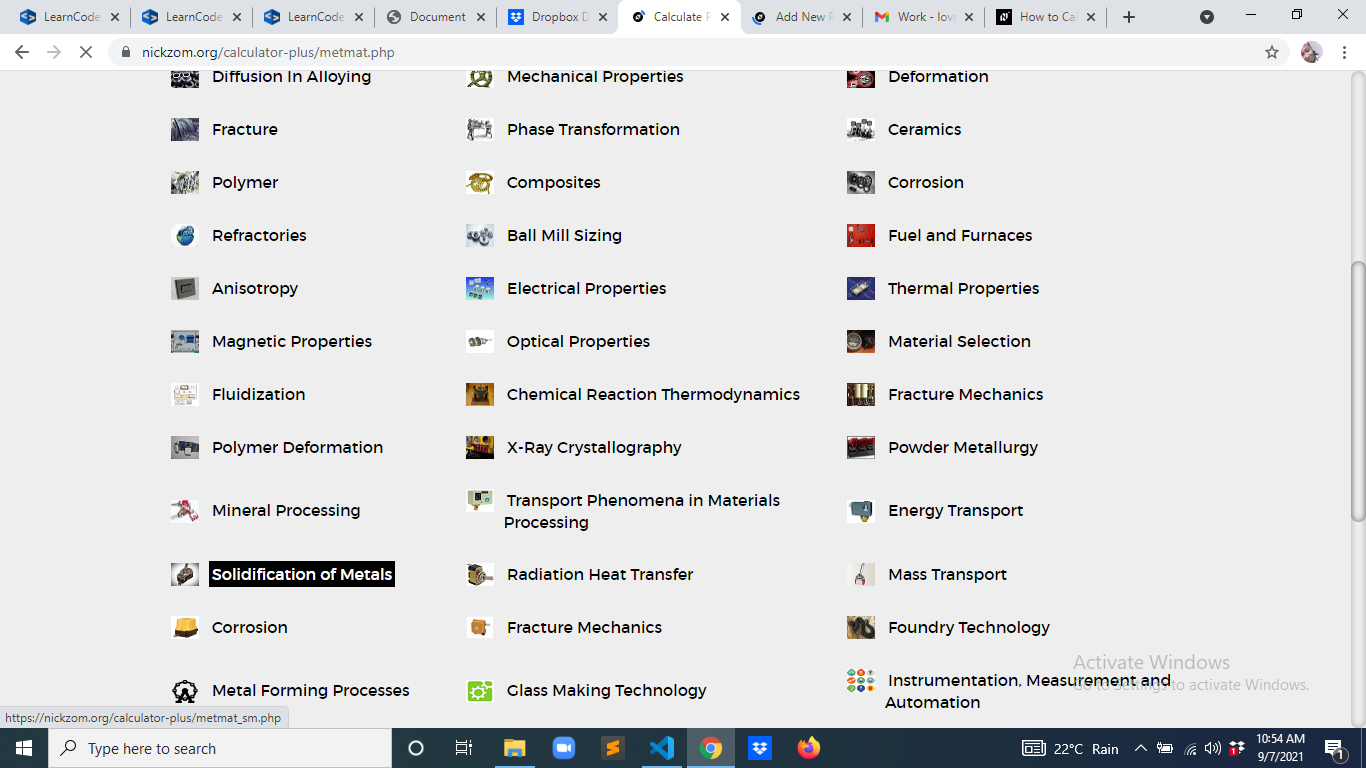Now, Click on Thickness of Solidifying Metals under Solidification of Metal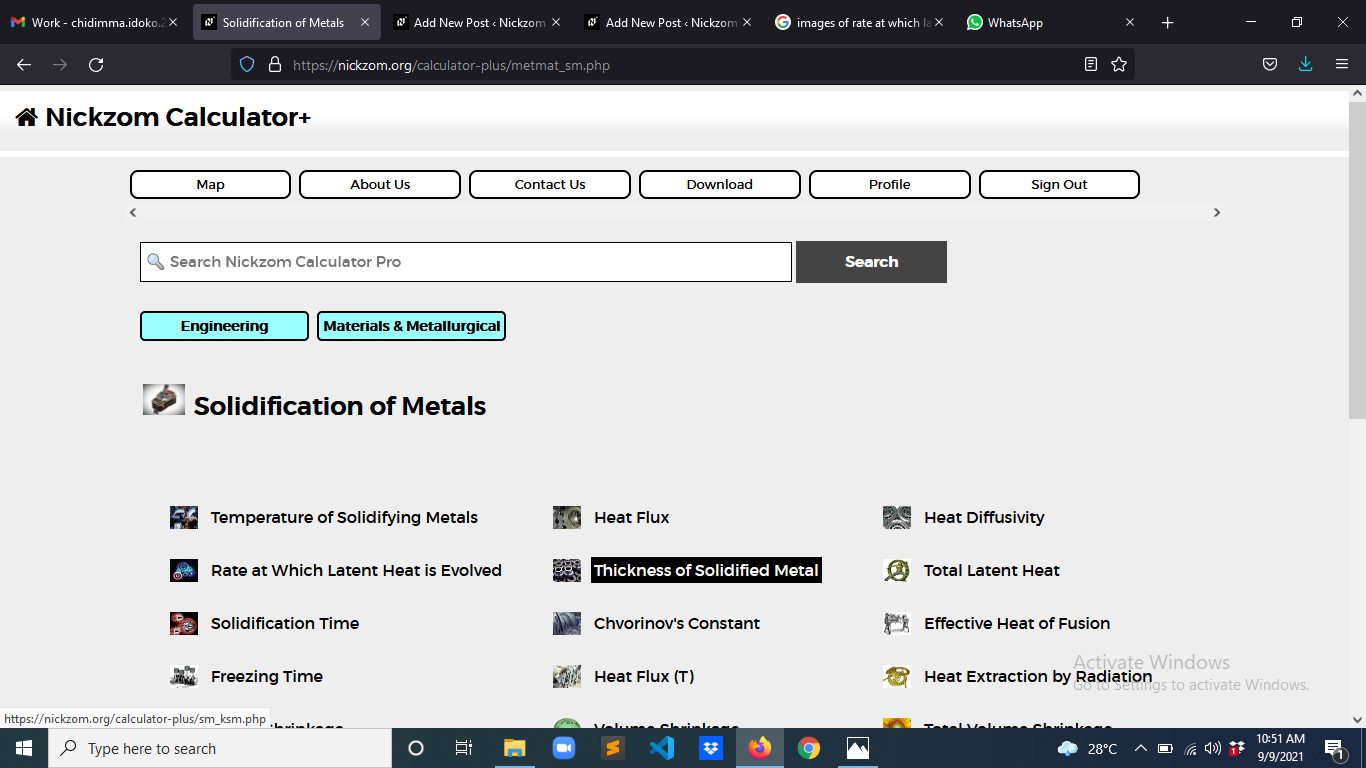The screenshot below displays the page or activity to enter your values, to get the answer for the thickness of solidifying metals according to the respective parameter which is the Melting Temperature of Metal (Tm), Initial Mould Temperature (To), Heat Diffusivity (α), Time (t), Density (ρ’) and Latent Heat of Fusion (Hf).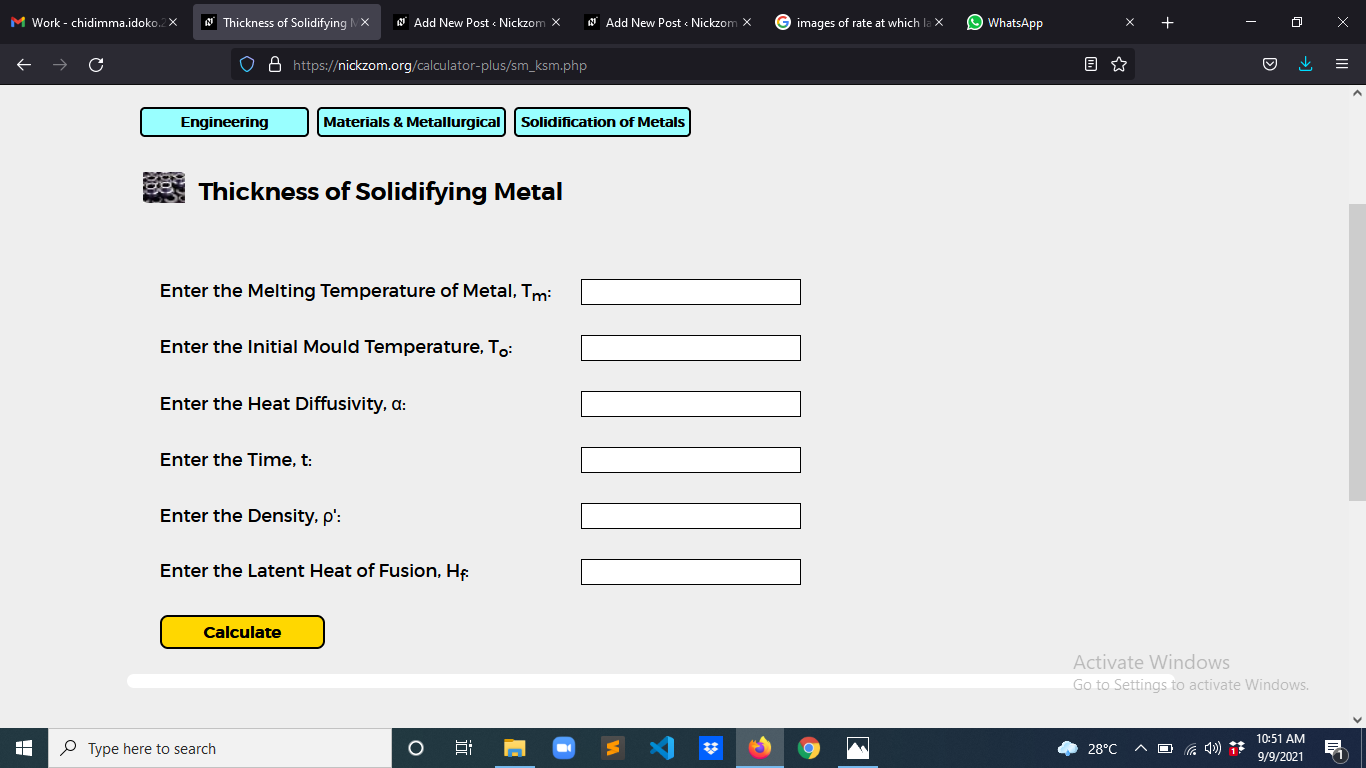Now, enter the values appropriately and accordingly for the parameters as required by the Melting Temperature of Metal (Tm) is 4, Initial Mould Temperature (To) is 8, Heat Diffusivity (α) is 2, Time (t) is 6, Density (ρ’) is 3 and Latent Heat of Fusion (Hf) is 7.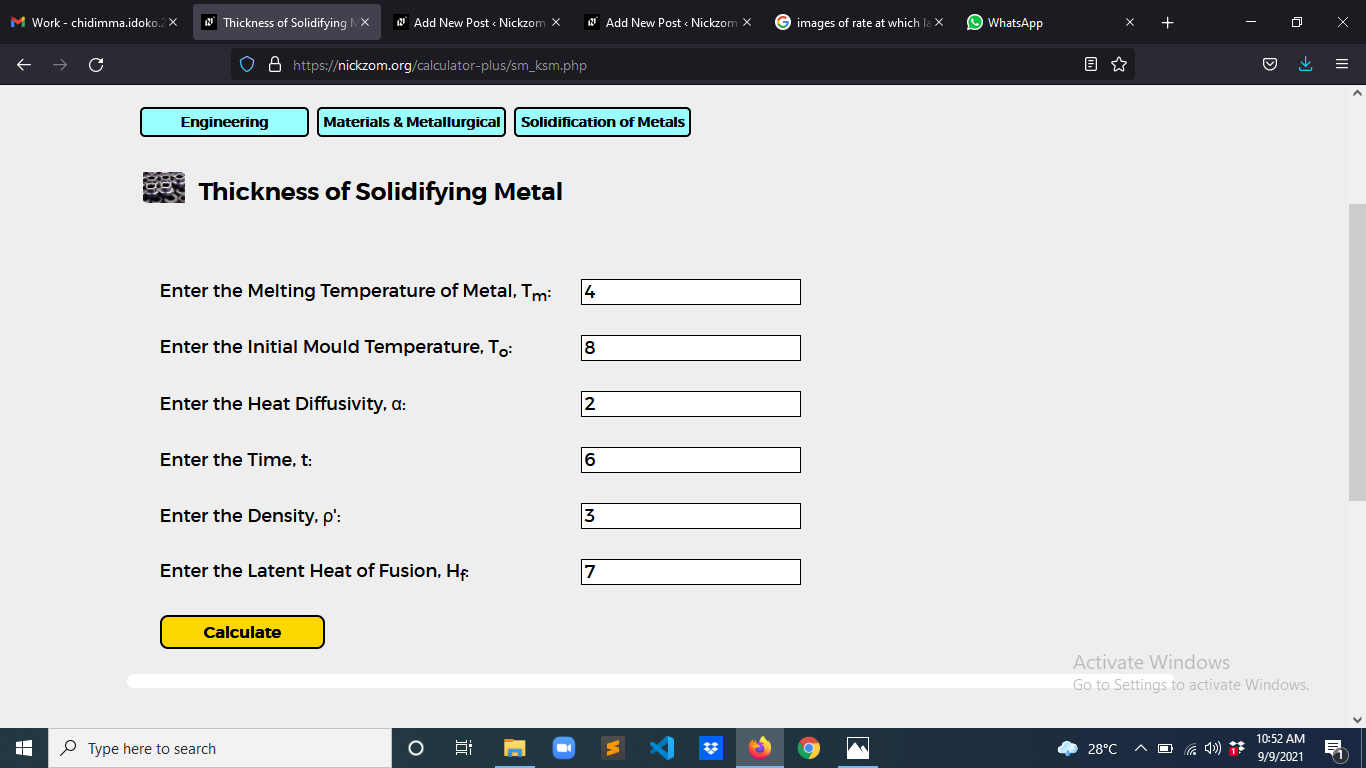Finally, Click on Calculate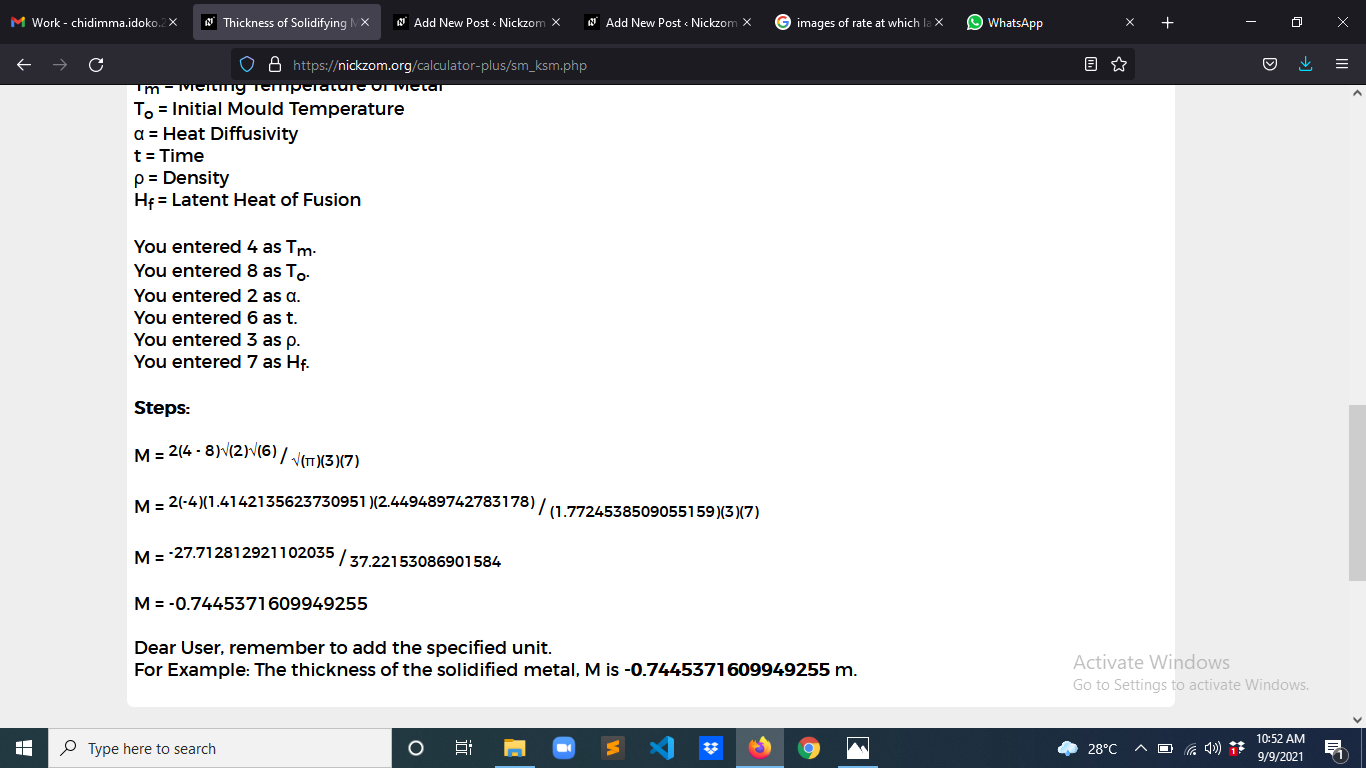As you can see from the screenshot above, Nickzom Calculator– The Calculator Encyclopedia solves for the thickness of solidifying metals and presents the formula, workings and steps too.